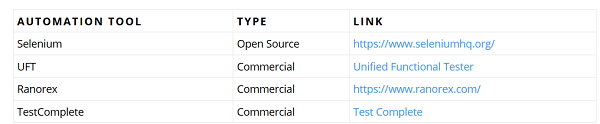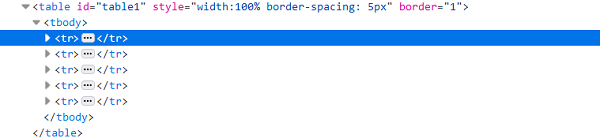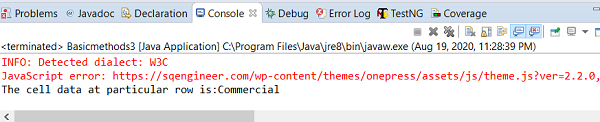# How to find specific lines in a table using Selenium?

We can find specific lines in a table with Selenium webdriver. A table is identified in an html document with <table> tag. Each table comprises a <tr> tag that represents rows and a <td> tag to represent columns. To traverse through table rows and columns we use the method findElements().

To count the total number of rows in table we use size() method available for list −

List <WebElement> l=driver.findElements(By.tagName("tr"));
int s= l.size();

To count the total number of columns in table we use size() method available for list −

List <WebElement> m=driver.findElements(By.tagName("td"));
int t= m.size();

Let us consider the below table and get the value of TYPE having AUTOMATION TOOL as UFT.The html structure of a table is described below −## Example

Code Implementation.

import org.openqa.selenium.By;
import org.openqa.selenium.WebDriver;
import org.openqa.selenium.WebElement;
import org.openqa.selenium.chrome.ChromeDriver;
import java.util.concurrent.TimeUnit;
public class TableHandling{
public static void main(String[] args) {
System.setProperty("webdriver.chrome.driver", "C:\Users\ghs6kor\Desktop\Java\chromedriver.exe");
WebDriver driver = new ChromeDriver();
String url = " https://sqengineer.com/practice-sites/practice-tables- selenium/";
driver.get(url);
driver.manage().timeouts().implicitlyWait(5, TimeUnit.SECONDS);
// identify table
WebElement mytable = driver.findElement(By.xpath("//table[@id='table1']/tbody"));
//identify rows of table.
List<WebElement> r_table = mytable.findElements(By.tagName("tr"));
String b_xpath = "//table[@id='table1']/tbody/tr[";
String a_xpath = "]/td";
//calculate rows number with size()
int rs_c = r_table.size();
//iterate rows of table and check matching condition
for (int r = 2;r <= rs_c; r++) {
String n = driver.findElement(By.xpath(b_xpath + r + a_xpath)).getText();
if(n.contains("UFT")){
// get text of matching cell
String                   celtxt=driver.findElement(By.xpath("//table[@id='table1']/tbody/tr["+r+"]/td")).getText();
System.out.println("The cell data at particular row is:" + celtxt);
break;
}
}
driver.quit();
}
}

## Output